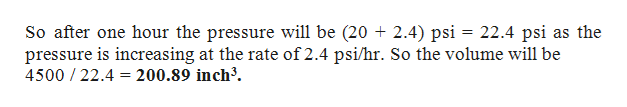# The volume of a balloon (V) and the pressure on that balloon (P) are related by the equation: PV=4500 (Boyle's Law). At a particular moment in time, the pressure on the balloon is 20 psi (pounds per square inch) and is increasing at the rate of 2.4 psi/hour. At what rate is the volume (measured in in^3) changing at that time? Label your answer with the appropriate units)

Question
30 views

The volume of a balloon (V) and the pressure on that balloon (P) are related by the equation: PV=4500 (Boyle's Law). At a particular moment in time, the pressure on the balloon is 20 psi (pounds per square inch) and is increasing at the rate of 2.4 psi/hour. At what rate is the volume (measured in in^3) changing at that time? Label your answer with the appropriate units)

check_circle

Step 1help_outlineImage TranscriptioncloseAt a particular moment in time, the pressure on the balloonis 20 psi. The PV relationship equation is given as Pv = 4500. Thus, the volume at that time = 4500 /20 =225 inch3. fullscreen
Step 2help_outlineImage TranscriptioncloseSo after one hour the pressure will be (20 2.4) psi 22.4 psi as the pressure is increasing at the rate of 2.4 psi/hr. So the volume will be 4500 / 22.4 200.89 inch3. fullscreen

### Want to see the full answer?

See Solution

#### Want to see this answer and more?

Solutions are written by subject experts who are available 24/7. Questions are typically answered within 1 hour.*

See Solution
*Response times may vary by subject and question.
Tagged in

### Physical Chemistry